# RD Sharma Solutions for Class 7 Maths Chapter 12 Profit And Loss

The PDF of RD Sharma Solutions for Class 7 Maths Chapter 12 Profit and Loss are downloaded from the given links. Class 7 is a stage where several new topics are introduced. Our experts formulate RD Sharma Solutions to help students in their exam preparation to achieve excellent marks in Maths. The solutions are stepwise and detailed to make learning easy for students. RD Sharma Solutions for Class 7 help students to get a good score in the examinations. It also provides extensive knowledge about the subject, as Class 7 builds a foundation in their academic career. Let us have a look at some important topics being discussed in this Chapter.

• Definition and meaning of profit and loss
• Definition and meaning of cost price
• Definition and meaning of selling price
• Profit percent and loss percent
• On finding profit or loss when C.P. and S.P. are given
• On computing C.P. or S.P. when profit or loss is given
• On finding profit or loss percent
• On finding the S.P. when C.P. and profit or loss percent are given
• On finding the cost price when S.P. and profit or loss are given

## Download the PDF of RD Sharma Solutions For Class 7 Maths Chapter 12 Profit And Loss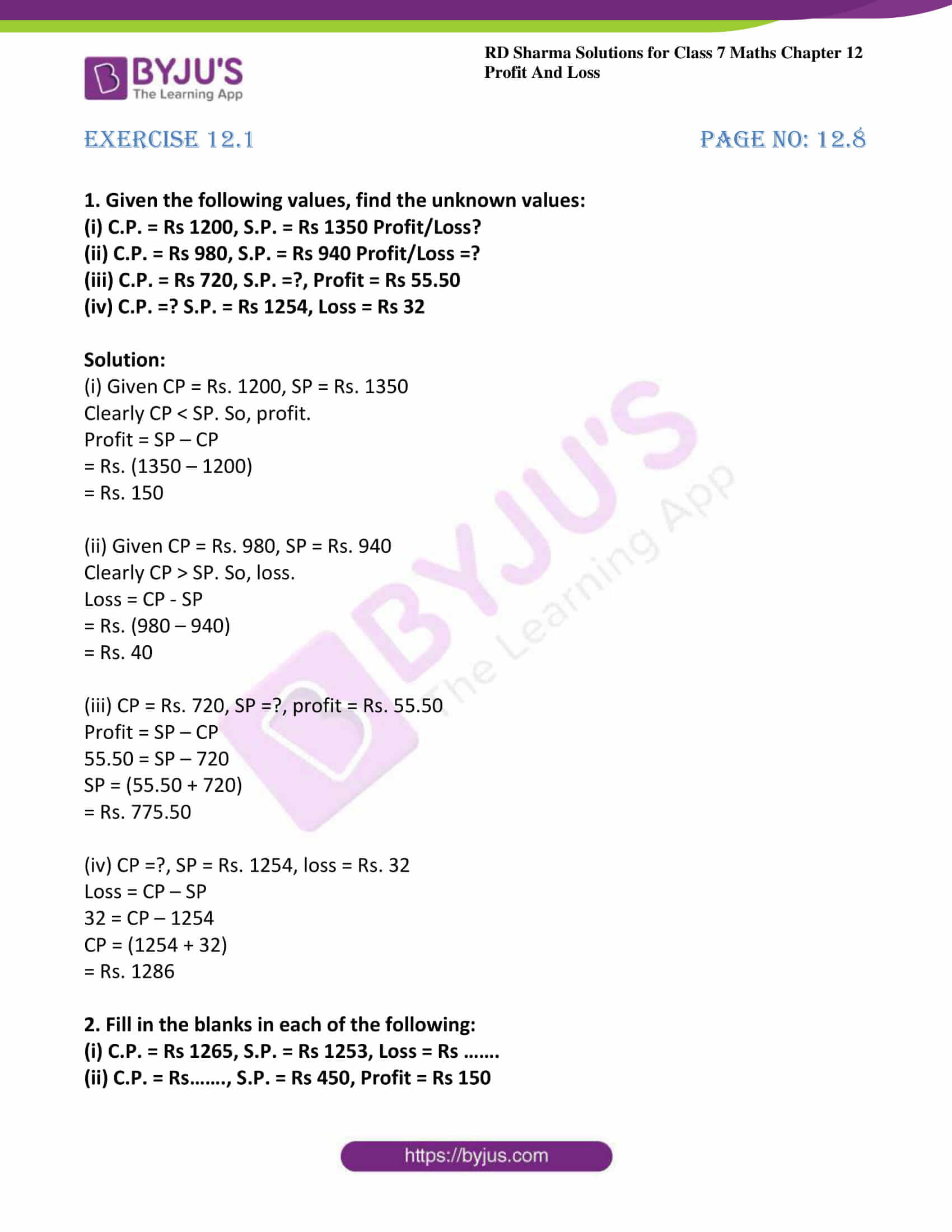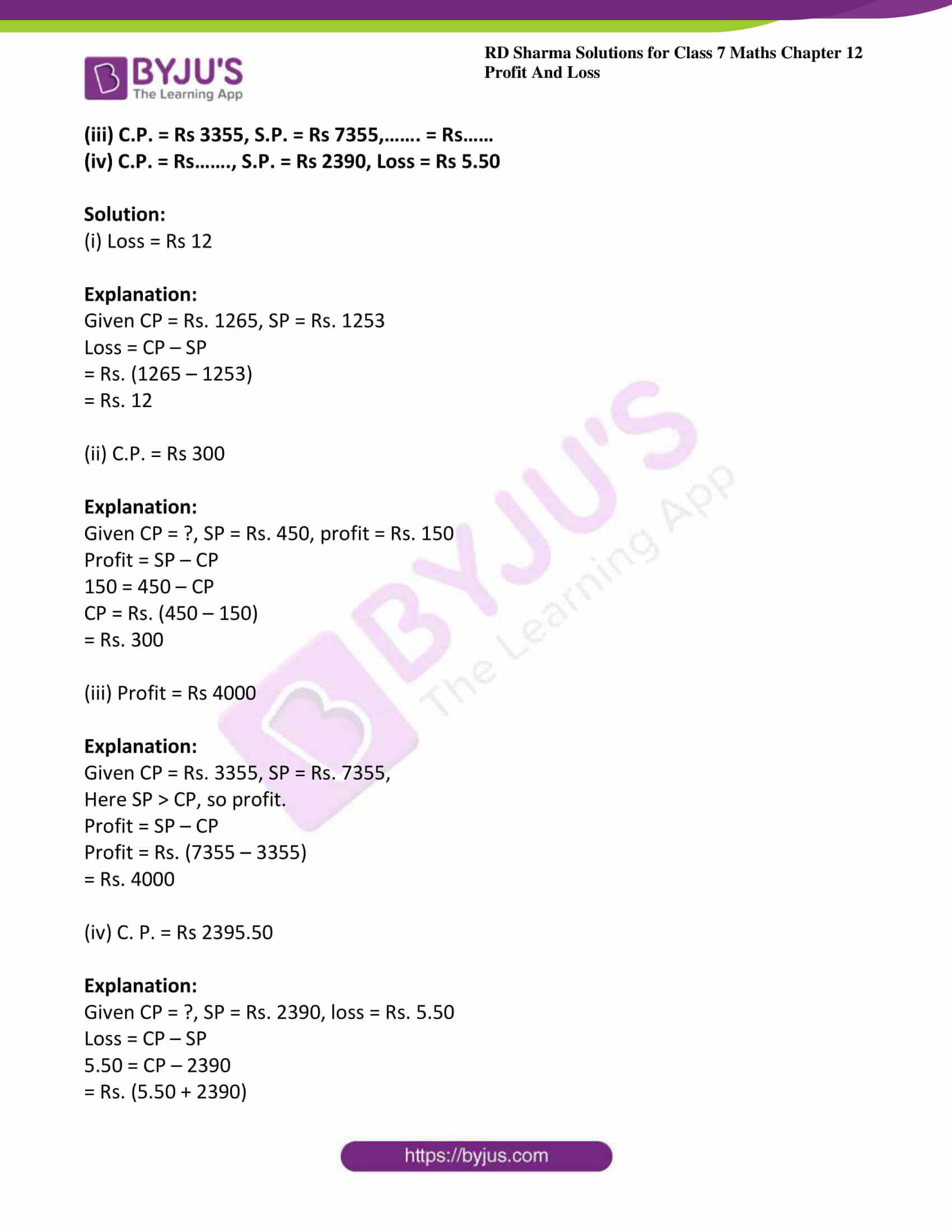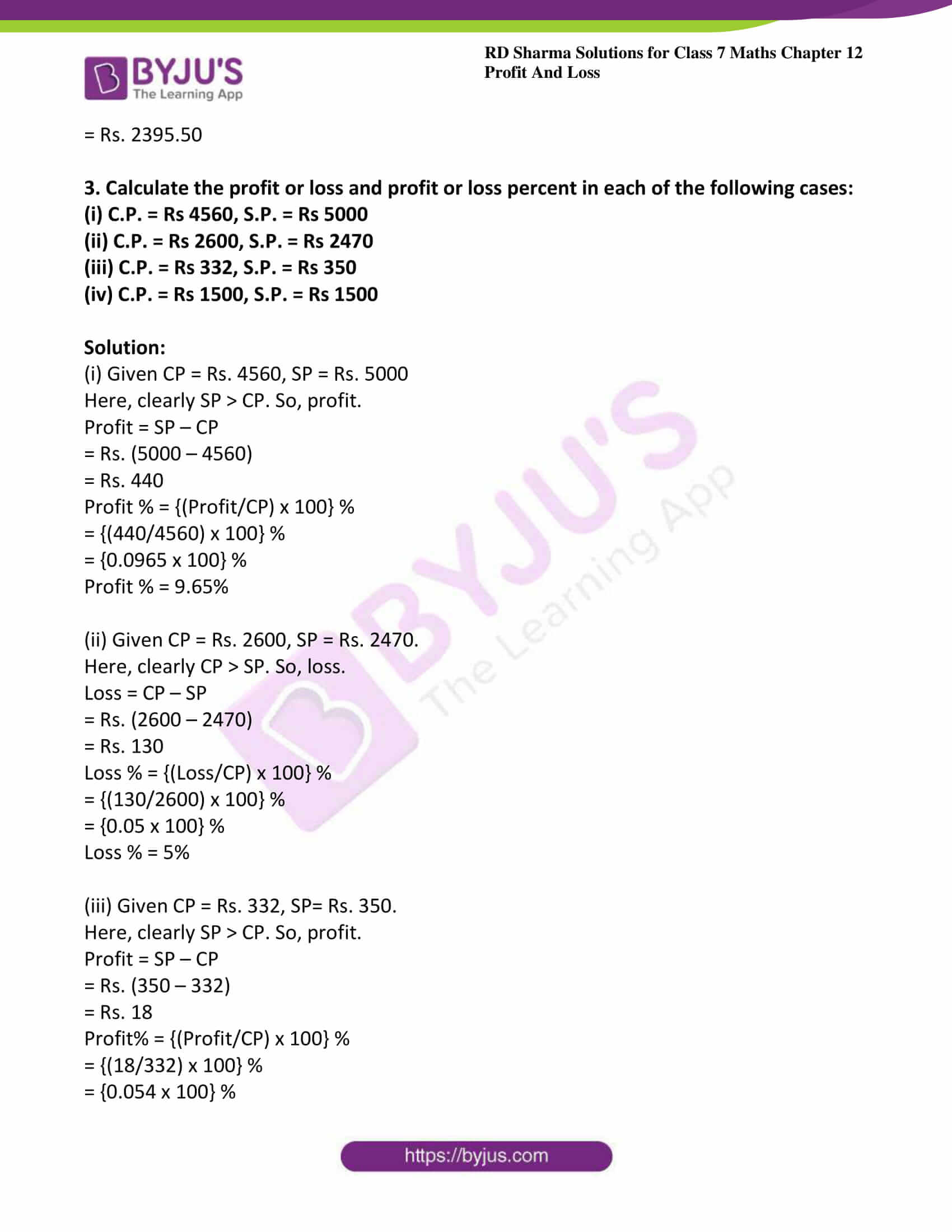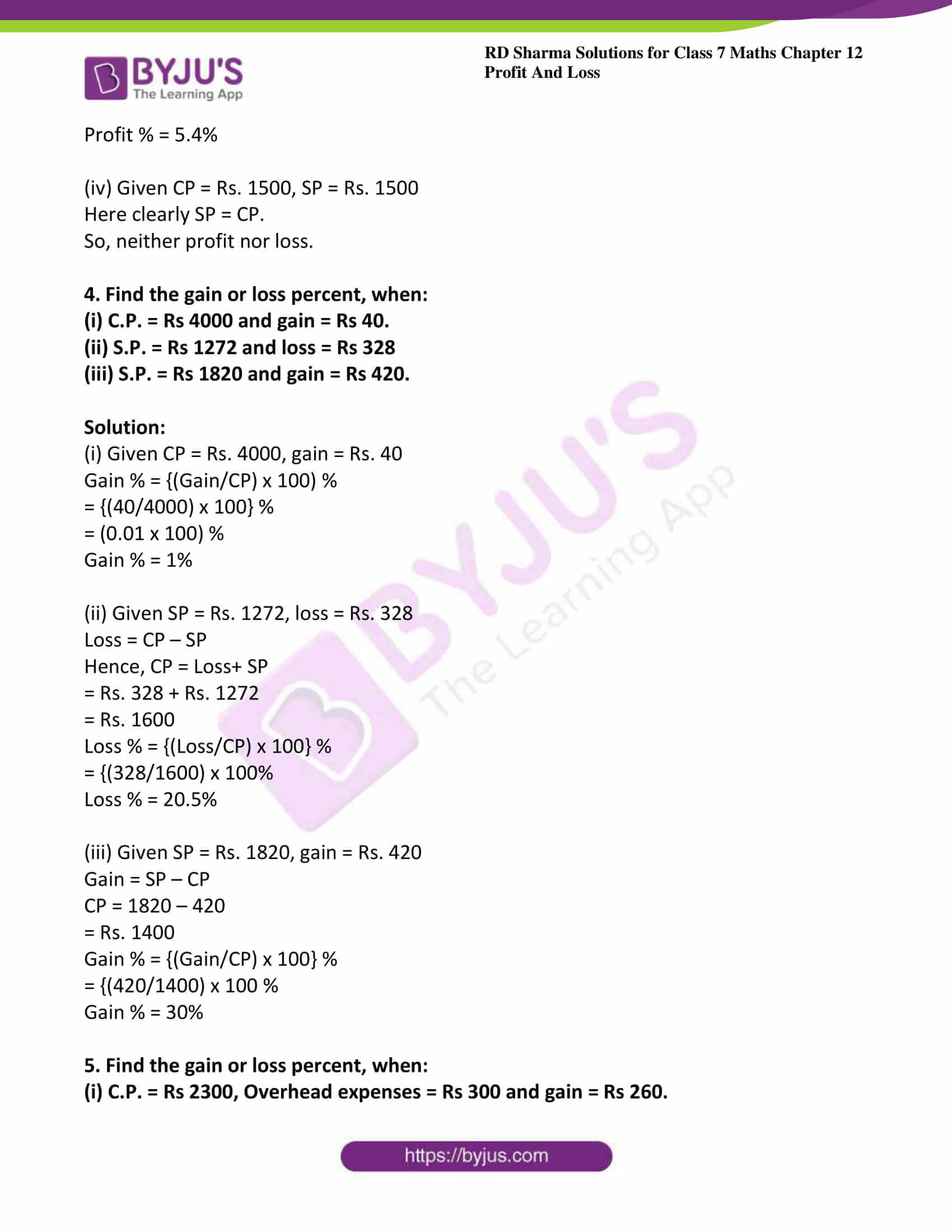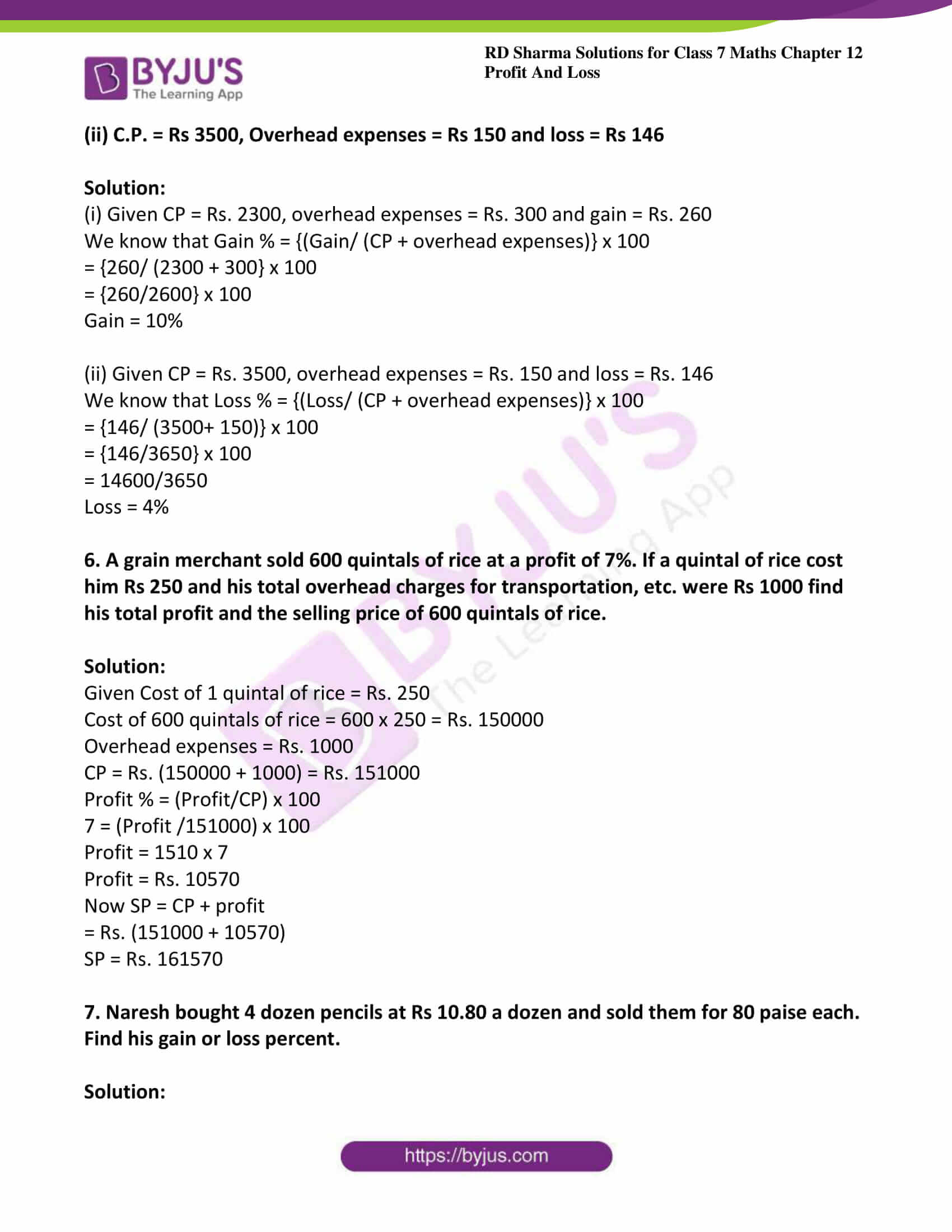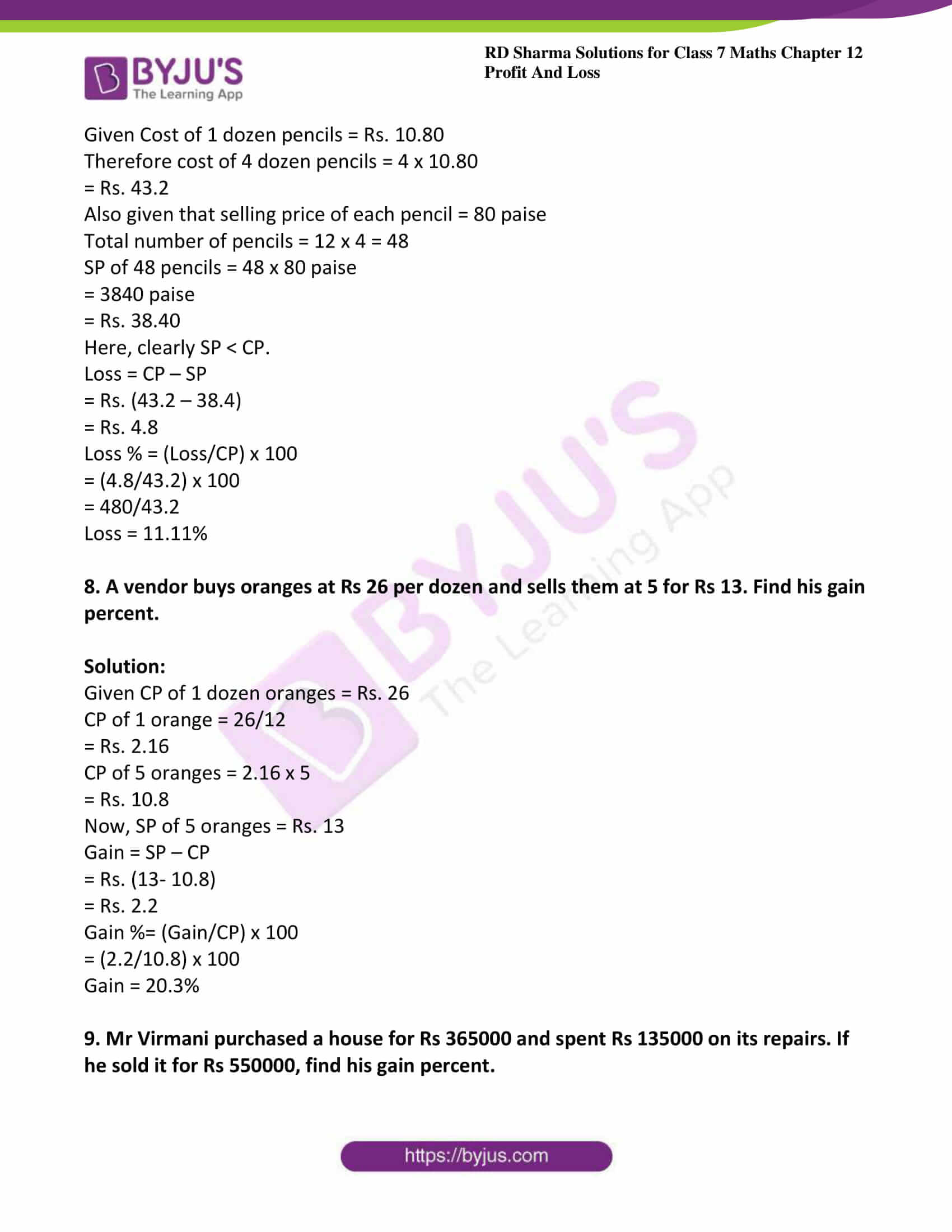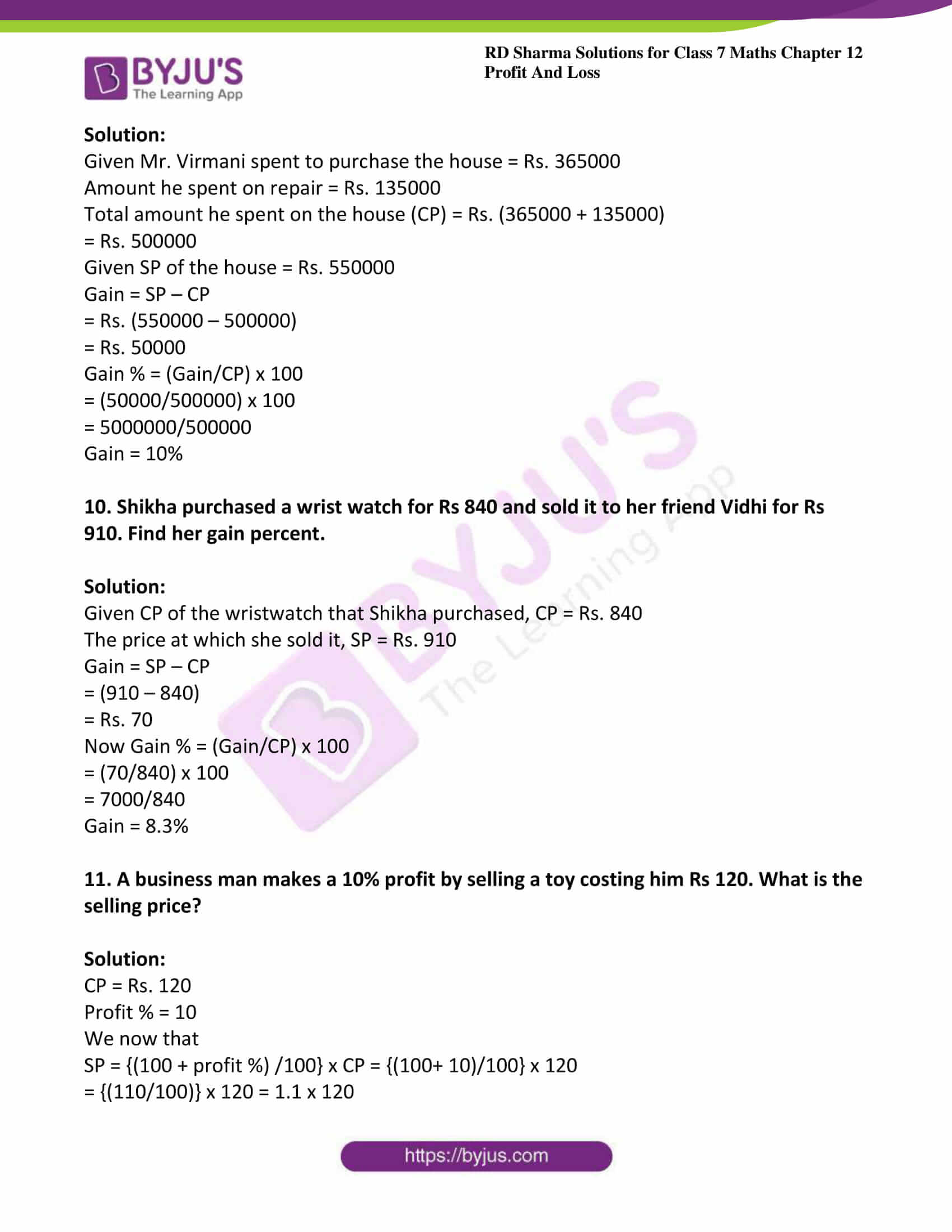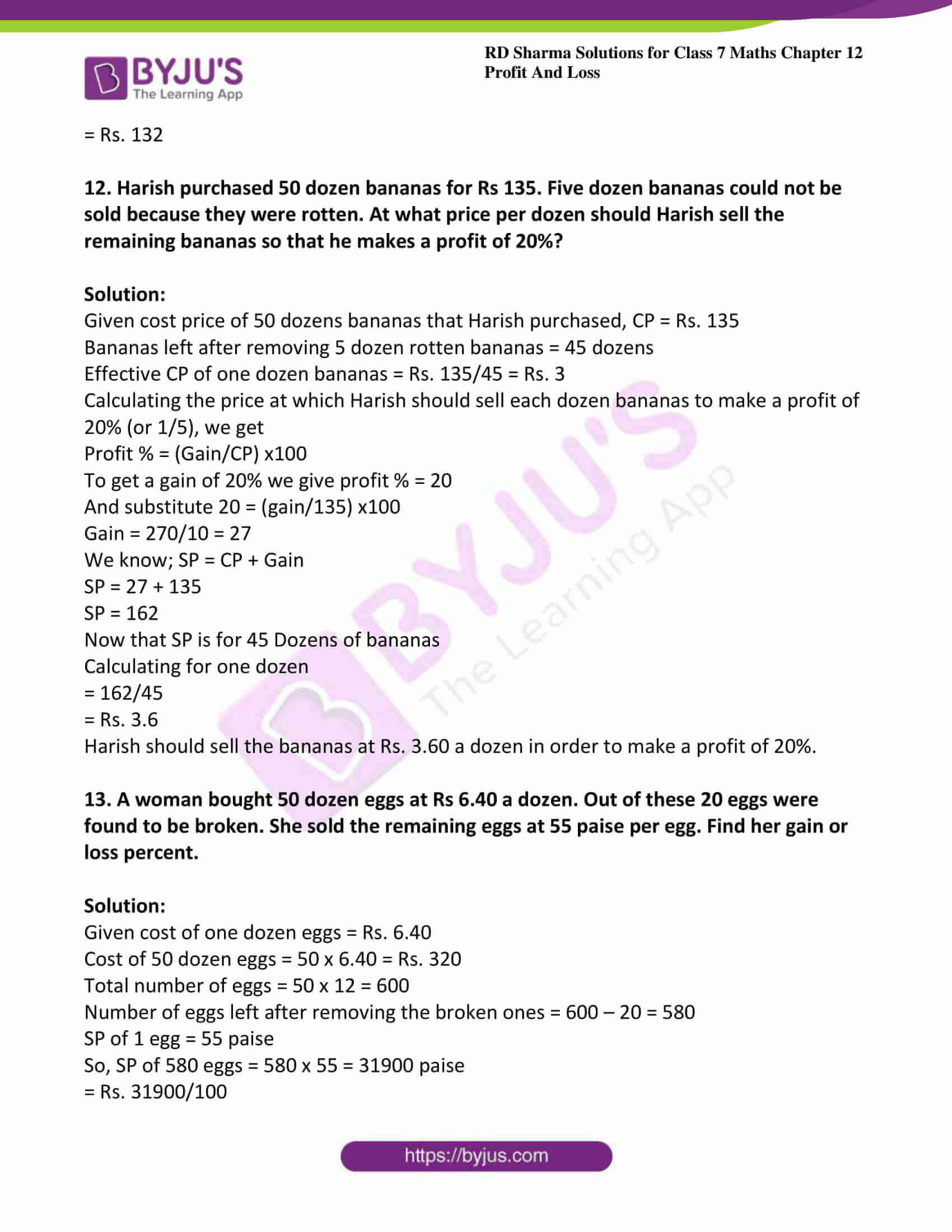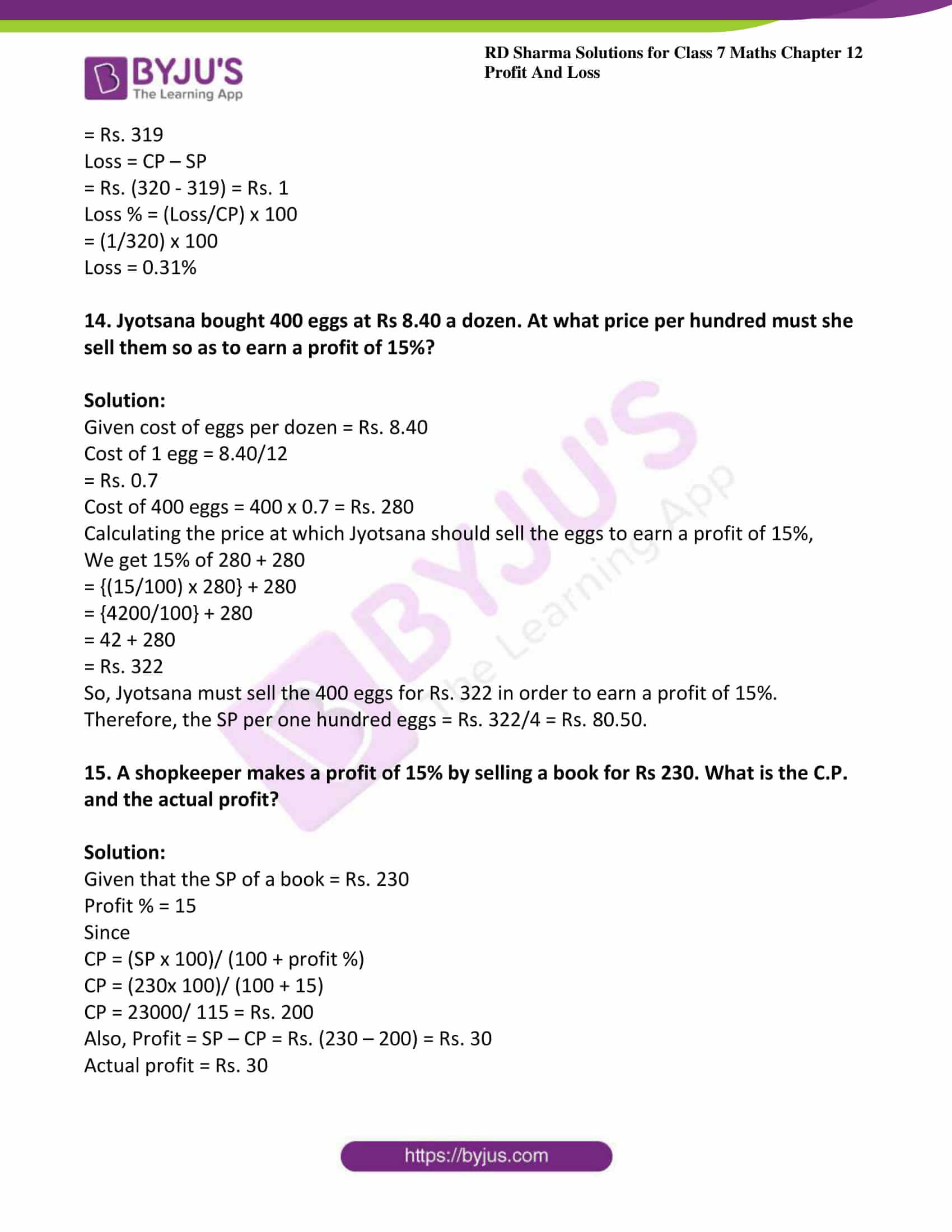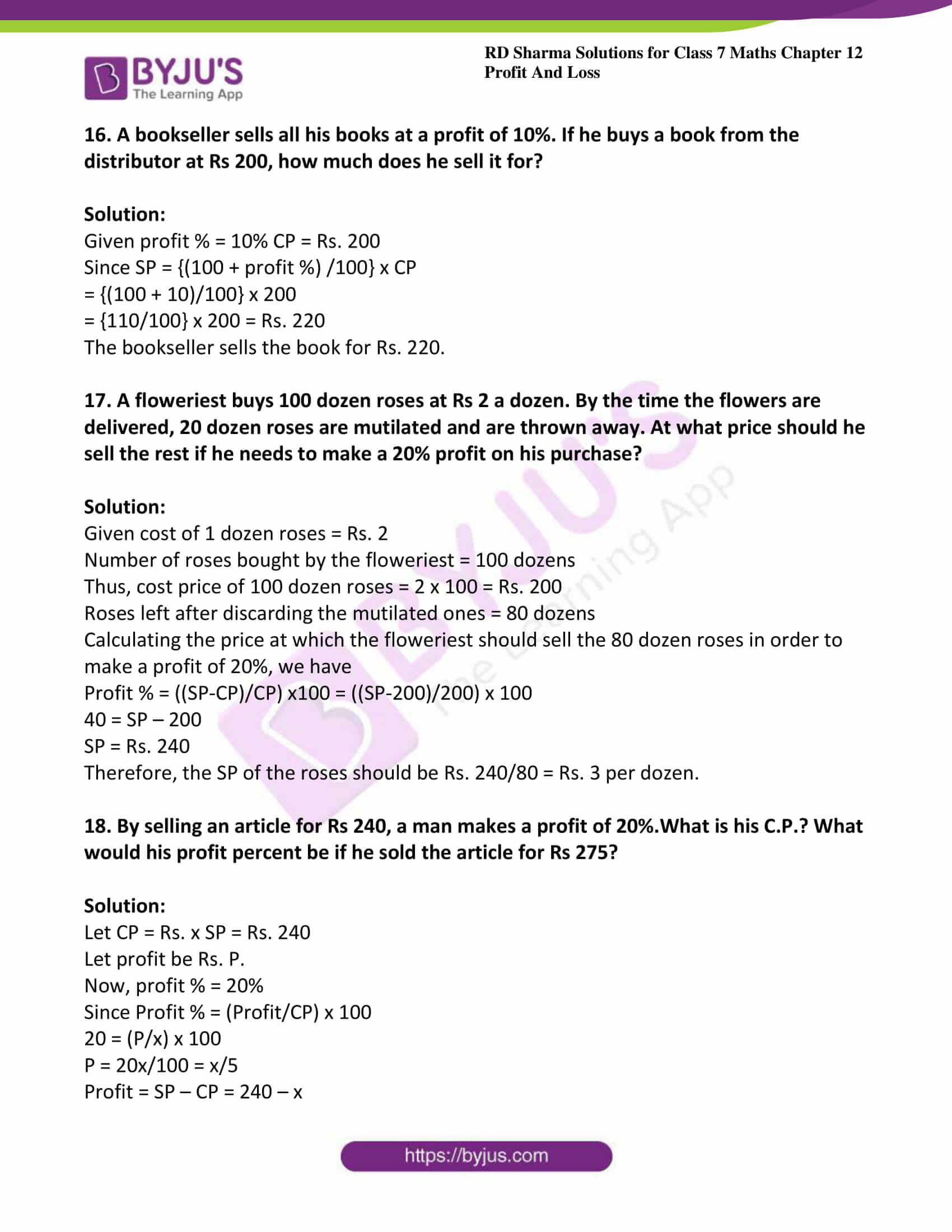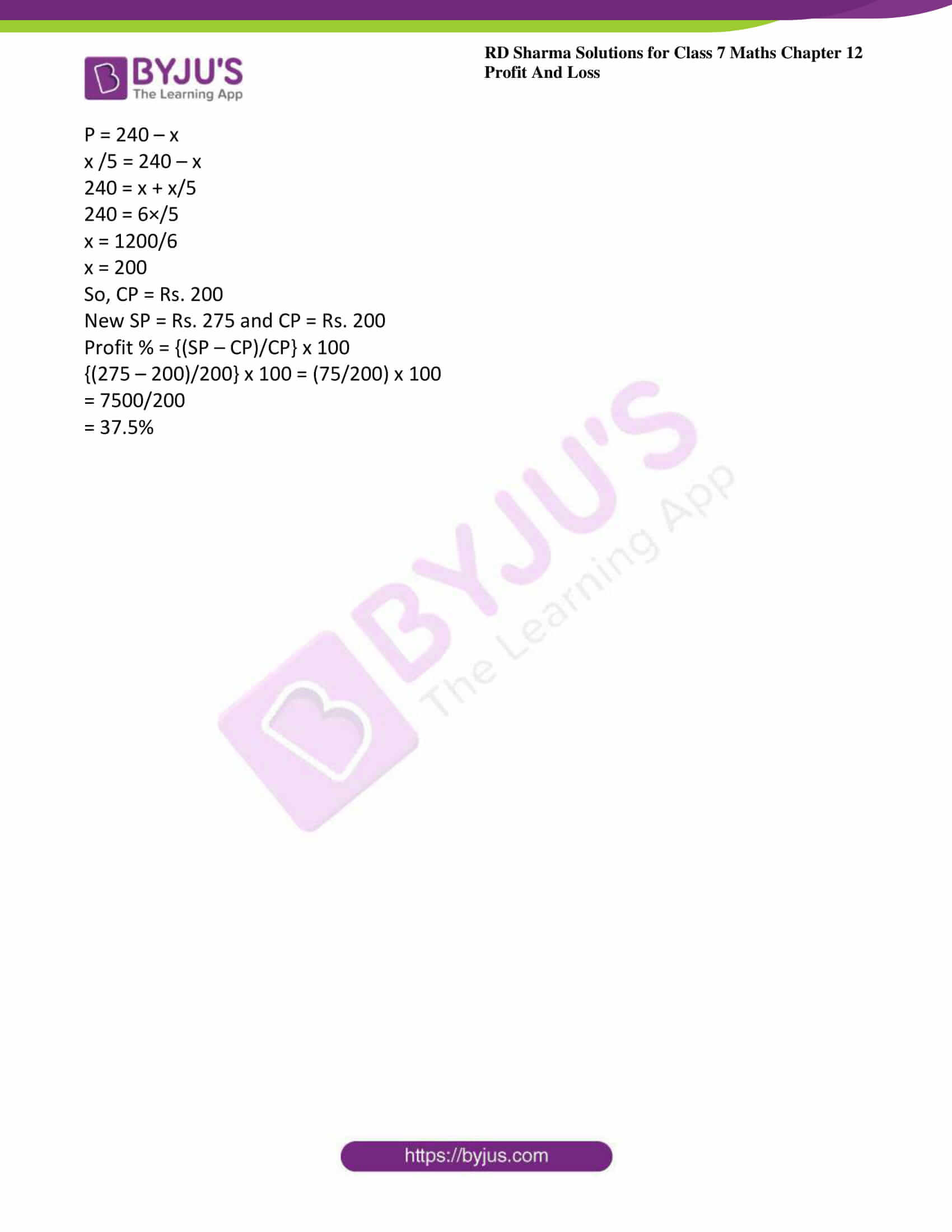### Access answers to Maths RD Sharma Solutions For Class 7 Chapter 12 – Profit And Loss

Exercise 12.1 Page No: 12.8

1. Given the following values, find the unknown values:

(i) C.P. = Rs 1200, S.P. = Rs 1350 Profit/Loss?

(ii) C.P. = Rs 980, S.P. = Rs 940 Profit/Loss =?

(iii) C.P. = Rs 720, S.P. =?, Profit = Rs 55.50

(iv) C.P. =? S.P. = Rs 1254, Loss = Rs 32

Solution:

(i) Given CP = Rs. 1200, SP = Rs. 1350

Clearly CP < SP. So, profit.

Profit = SP – CP

= Rs. (1350 – 1200)

= Rs. 150

(ii) Given CP = Rs. 980, SP = Rs. 940

Clearly CP > SP. So, loss.

Loss = CP – SP

= Rs. (980 – 940)

= Rs. 40

(iii) CP = Rs. 720, SP =?, profit = Rs. 55.50

Profit = SP – CP

55.50 = SP – 720

SP = (55.50 + 720)

= Rs. 775.50

(iv) CP =?, SP = Rs. 1254, loss = Rs. 32

Loss = CP – SP

32 = CP – 1254

CP = (1254 + 32)

= Rs. 1286

2. Fill in the blanks in each of the following:

(i) C.P. = Rs 1265, S.P. = Rs 1253, Loss = Rs …….

(ii) C.P. = Rs……., S.P. = Rs 450, Profit = Rs 150

(iii) C.P. = Rs 3355, S.P. = Rs 7355,……. = Rs……

(iv) C.P. = Rs……., S.P. = Rs 2390, Loss = Rs 5.50

Solution:

(i) Loss = Rs 12

Explanation:

Given CP = Rs. 1265, SP = Rs. 1253

Loss = CP – SP

= Rs. (1265 – 1253)

= Rs. 12

(ii) C.P. = Rs 300

Explanation:

Given CP = ?, SP = Rs. 450, profit = Rs. 150

Profit = SP – CP

150 = 450 – CP

CP = Rs. (450 – 150)

= Rs. 300

(iii) Profit = Rs 4000

Explanation:

Given CP = Rs. 3355, SP = Rs. 7355,

Here SP > CP, so profit.

Profit = SP – CP

Profit = Rs. (7355 – 3355)

= Rs. 4000

(iv) C. P. = Rs 2395.50

Explanation:

Given CP = ?, SP = Rs. 2390, loss = Rs. 5.50

Loss = CP – SP

5.50 = CP – 2390

= Rs. (5.50 + 2390)

= Rs. 2395.50

3. Calculate the profit or loss and profit or loss percent in each of the following cases:

(i) C.P. = Rs 4560, S.P. = Rs 5000

(ii) C.P. = Rs 2600, S.P. = Rs 2470

(iii) C.P. = Rs 332, S.P. = Rs 350

(iv) C.P. = Rs 1500, S.P. = Rs 1500

Solution:

(i) Given CP = Rs. 4560, SP = Rs. 5000

Here, clearly SP > CP. So, profit.

Profit = SP – CP

= Rs. (5000 – 4560)

= Rs. 440

Profit % = {(Profit/CP) x 100} %

= {(440/4560) x 100} %

= {0.0965 x 100} %

Profit % = 9.65%

(ii) Given CP = Rs. 2600, SP = Rs. 2470.

Here, clearly CP > SP. So, loss.

Loss = CP – SP

= Rs. (2600 – 2470)

= Rs. 130

Loss % = {(Loss/CP) x 100} %

= {(130/2600) x 100} %

= {0.05 x 100} %

Loss % = 5%

(iii) Given CP = Rs. 332, SP= Rs. 350.

Here, clearly SP > CP. So, profit.

Profit = SP – CP

= Rs. (350 – 332)

= Rs. 18

Profit% = {(Profit/CP) x 100} %

= {(18/332) x 100} %

= {0.054 x 100} %

Profit % = 5.4%

(iv) Given CP = Rs. 1500, SP = Rs. 1500

Here clearly SP = CP.

So, neither profit nor loss.

4. Find the gain or loss percent, when:

(i) C.P. = Rs 4000 and gain = Rs 40.

(ii) S.P. = Rs 1272 and loss = Rs 328

(iii) S.P. = Rs 1820 and gain = Rs 420.

Solution:

(i) Given CP = Rs. 4000, gain = Rs. 40

Gain % = {(Gain/CP) x 100) %

= {(40/4000) x 100} %

= (0.01 x 100) %

Gain % = 1%

(ii) Given SP = Rs. 1272, loss = Rs. 328

Loss = CP – SP

Hence, CP = Loss+ SP

= Rs. 328 + Rs. 1272

= Rs. 1600

Loss % = {(Loss/CP) x 100} %

= {(328/1600) x 100%

Loss % = 20.5%

(iii) Given SP = Rs. 1820, gain = Rs. 420

Gain = SP – CP

CP = 1820 – 420

= Rs. 1400

Gain % = {(Gain/CP) x 100} %

= {(420/1400) x 100 %

Gain % = 30%

5. Find the gain or loss percent, when:

(i) C.P. = Rs 2300, Overhead expenses = Rs 300 and gain = Rs 260.

(ii) C.P. = Rs 3500, Overhead expenses = Rs 150 and loss = Rs 146

Solution:

(i) Given CP = Rs. 2300, overhead expenses = Rs. 300 and gain = Rs. 260

We know that Gain % = {(Gain/ (CP + overhead expenses)} x 100

= {260/ (2300 + 300} x 100

= {260/2600} x 100

Gain = 10%

(ii) Given CP = Rs. 3500, overhead expenses = Rs. 150 and loss = Rs. 146

We know that Loss % = {(Loss/ (CP + overhead expenses)} x 100

= {146/ (3500+ 150)} x 100

= {146/3650} x 100

= 14600/3650

Loss = 4%

6. A grain merchant sold 600 quintals of rice at a profit of 7%. If a quintal of rice cost him Rs 250 and his total overhead charges for transportation, etc. were Rs 1000 find his total profit and the selling price of 600 quintals of rice.

Solution:

Given Cost of 1 quintal of rice = Rs. 250

Cost of 600 quintals of rice = 600 x 250 = Rs. 150000

CP = Rs. (150000 + 1000) = Rs. 151000

Profit % = (Profit/CP) x 100

7 = (Profit /151000) x 100

Profit = 1510 x 7

Profit = Rs. 10570

Now SP = CP + profit

= Rs. (151000 + 10570)

SP = Rs. 161570

7. Naresh bought 4 dozen pencils at Rs 10.80 a dozen and sold them for 80 paise each. Find his gain or loss percent.

Solution:

Given Cost of 1 dozen pencils = Rs. 10.80

Therefore cost of 4 dozen pencils = 4 x 10.80

= Rs. 43.2

Also given that selling price of each pencil = 80 paise

Total number of pencils = 12 x 4 = 48

SP of 48 pencils = 48 x 80 paise

= 3840 paise

= Rs. 38.40

Here, clearly SP < CP.

Loss = CP – SP

= Rs. (43.2 – 38.4)

= Rs. 4.8

Loss % = (Loss/CP) x 100

= (4.8/43.2) x 100

= 480/43.2

Loss = 11.11%

8. A vendor buys oranges at Rs 26 per dozen and sells them at 5 for Rs 13. Find his gain percent.

Solution:

Given CP of 1 dozen oranges = Rs. 26

CP of 1 orange = 26/12

= Rs. 2.16

CP of 5 oranges = 2.16 x 5

= Rs. 10.8

Now, SP of 5 oranges = Rs. 13

Gain = SP – CP

= Rs. (13- 10.8)

= Rs. 2.2

Gain %= (Gain/CP) x 100

= (2.2/10.8) x 100

Gain = 20.3%

9. Mr Virmani purchased a house for Rs 365000 and spent Rs 135000 on its repairs. If he sold it for Rs 550000, find his gain percent.

Solution:

Given Mr. Virmani spent to purchase the house = Rs. 365000

Amount he spent on repair = Rs. 135000

Total amount he spent on the house (CP) = Rs. (365000 + 135000)

= Rs. 500000

Given SP of the house = Rs. 550000

Gain = SP – CP

= Rs. (550000 – 500000)

= Rs. 50000

Gain % = (Gain/CP) x 100

= (50000/500000) x 100

= 5000000/500000

Gain = 10%

10. Shikha purchased a wrist watch for Rs 840 and sold it to her friend Vidhi for Rs 910. Find her gain percent.

Solution:

Given CP of the wristwatch that Shikha purchased, CP = Rs. 840

The price at which she sold it, SP = Rs. 910

Gain = SP – CP

= (910 – 840)

= Rs. 70

Now Gain % = (Gain/CP) x 100

= (70/840) x 100

= 7000/840

Gain = 8.3%

11. A business man makes a 10% profit by selling a toy costing him Rs 120. What is the selling price?

Solution:

CP = Rs. 120

Profit % = 10

We now that

SP = {(100 + profit %) /100} x CP = {(100+ 10)/100} x 120

= {(110/100)} x 120 = 1.1 x 120

= Rs. 132

12. Harish purchased 50 dozen bananas for Rs 135. Five dozen bananas could not be sold because they were rotten. At what price per dozen should Harish sell the remaining bananas so that he makes a profit of 20%?

Solution:

Given cost price of 50 dozens bananas that Harish purchased, CP = Rs. 135

Bananas left after removing 5 dozen rotten bananas = 45 dozens

Effective CP of one dozen bananas = Rs. 135/45 = Rs. 3

Calculating the price at which Harish should sell each dozen bananas to make a profit of 20% (or 1/5), we get

Profit % = (Gain/CP) x100

To get a gain of 20% we give profit % = 20

And substitute 20 = (gain/135) x100

Gain = 270/10 = 27

We know; SP = CP + Gain

SP = 27 + 135

SP = 162

Now that SP is for 45 Dozens of bananas

Calculating for one dozen

= 162/45

= Rs. 3.6

Harish should sell the bananas at Rs. 3.60 a dozen in order to make a profit of 20%.

13. A woman bought 50 dozen eggs at Rs 6.40 a dozen. Out of these 20 eggs were found to be broken. She sold the remaining eggs at 55 paise per egg. Find her gain or loss percent.

Solution:

Given cost of one dozen eggs = Rs. 6.40

Cost of 50 dozen eggs = 50 x 6.40 = Rs. 320

Total number of eggs = 50 x 12 = 600

Number of eggs left after removing the broken ones = 600 – 20 = 580

SP of 1 egg = 55 paise

So, SP of 580 eggs = 580 x 55 = 31900 paise

= Rs. 31900/100

= Rs. 319

Loss = CP – SP

= Rs. (320 – 319) = Rs. 1

Loss % = (Loss/CP) x 100

= (1/320) x 100

Loss = 0.31%

14. Jyotsana bought 400 eggs at Rs 8.40 a dozen. At what price per hundred must she sell them so as to earn a profit of 15%?

Solution:

Given cost of eggs per dozen = Rs. 8.40

Cost of 1 egg = 8.40/12

= Rs. 0.7

Cost of 400 eggs = 400 x 0.7 = Rs. 280

Calculating the price at which Jyotsana should sell the eggs to earn a profit of 15%,

We get 15% of 280 + 280

= {(15/100) x 280} + 280

= {4200/100} + 280

= 42 + 280

= Rs. 322

So, Jyotsana must sell the 400 eggs for Rs. 322 in order to earn a profit of 15%. Therefore, the SP per one hundred eggs = Rs. 322/4 = Rs. 80.50.

15. A shopkeeper makes a profit of 15% by selling a book for Rs 230. What is the C.P. and the actual profit?

Solution:

Given that the SP of a book = Rs. 230

Profit % = 15

Since

CP = (SP x 100)/ (100 + profit %)

CP = (230x 100)/ (100 + 15)

CP = 23000/ 115 = Rs. 200

Also, Profit = SP – CP = Rs. (230 – 200) = Rs. 30

Actual profit = Rs. 30

16. A bookseller sells all his books at a profit of 10%. If he buys a book from the distributor at Rs 200, how much does he sell it for?

Solution:

Given profit % = 10% CP = Rs. 200

Since SP = {(100 + profit %) /100} x CP

= {(100 + 10)/100} x 200

= {110/100} x 200 = Rs. 220

The bookseller sells the book for Rs. 220.

17. A floweriest buys 100 dozen roses at Rs 2 a dozen. By the time the flowers are delivered, 20 dozen roses are mutilated and are thrown away. At what price should he sell the rest if he needs to make a 20% profit on his purchase?

Solution:

Given cost of 1 dozen roses = Rs. 2

Number of roses bought by the floweriest = 100 dozens

Thus, cost price of 100 dozen roses = 2 x 100 = Rs. 200

Roses left after discarding the mutilated ones = 80 dozens

Calculating the price at which the floweriest should sell the 80 dozen roses in order to make a profit of 20%, we have

Profit % = ((SP-CP)/CP) x100 = ((SP-200)/200) x 100

40 = SP – 200

SP = Rs. 240

Therefore, the SP of the roses should be Rs. 240/80 = Rs. 3 per dozen.

18. By selling an article for Rs 240, a man makes a profit of 20%.What is his C.P.? What would his profit percent be if he sold the article for Rs 275?

Solution:

Let CP = Rs. x SP = Rs. 240

Let profit be Rs. P.

Now, profit % = 20%

Since Profit % = (Profit/CP) x 100

20 = (P/x) x 100

P = 20x/100 = x/5

Profit = SP – CP = 240 – x

P = 240 – x

x /5 = 240 – x

240 = x + x/5

240 = 6×/5

x = 1200/6

x = 200

So, CP = Rs. 200

New SP = Rs. 275 and CP = Rs. 200

Profit % = {(SP – CP)/CP} x 100

{(275 – 200)/200} x 100 = (75/200) x 100

= 7500/200

= 37.5%

1. Jai Prakash

Very nice